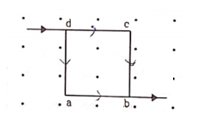# A current of 2A enters at the corner d of a square frame abcd of side 20cm

Question:

A current of $2 \mathrm{~A}$ enters at the corner $\mathrm{d}$ of a square frame abcd of side $20 \mathrm{~cm}$ and leaves at the opposite corner b. A magnetic field $B=0.1 \mathrm{~T}$ exists in the space in a direction perpendicular to the plane of the frame as shown in figure. Find the magnitude and direction of the magnetic forces on the four sides of the frame.Solution: